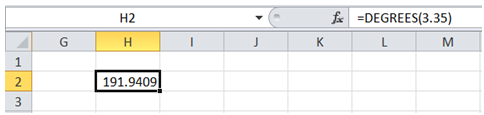## Convert radian into degree in Excel

For example, I want to convert 3.35 radians returned by one of my Engineering formulas to degrees so that I can make the necessary Engineering change.

To do it in Excel, here is the answer:

a) Enter the formula =DEGREES(number), where number is the radian value that has to be converted to degrees.